# Motivation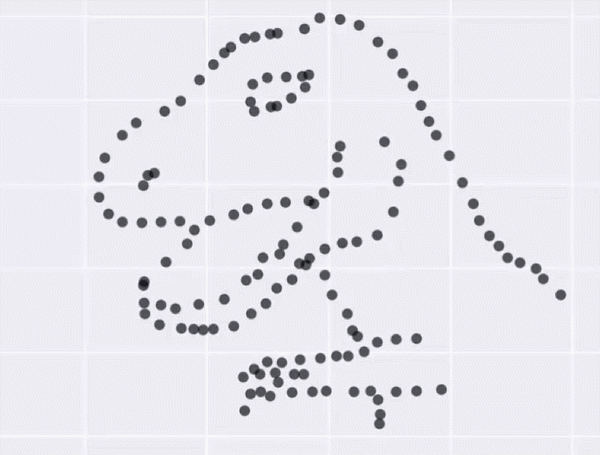The Datasaurus Dozen — What is common among these diverse datasets?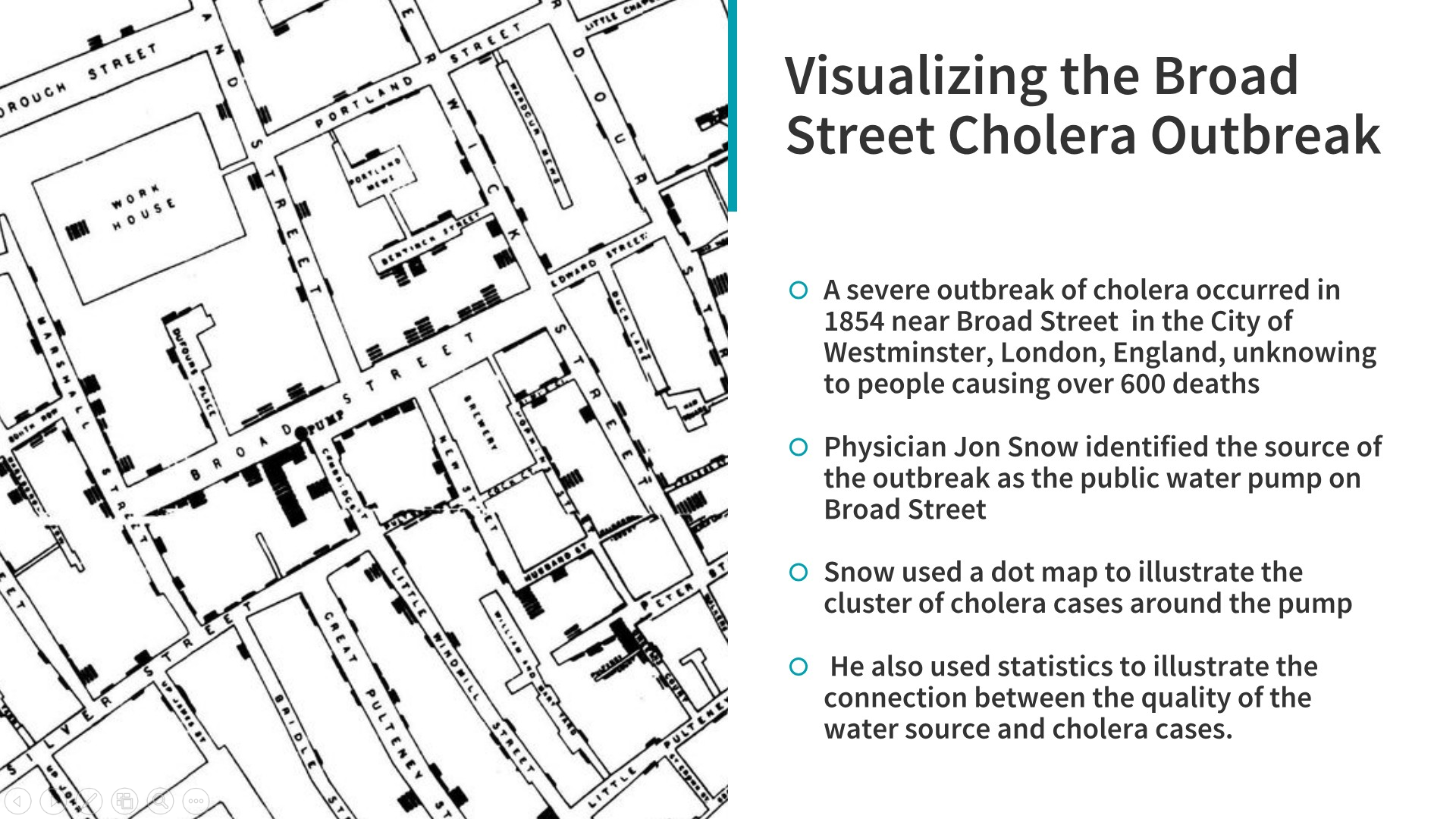Visualizing the Broad Street Cholera outbreak which helped find the root cause of the disease outbreak! (Source: https://github.com/dipanjanS/art_of_data_visualization)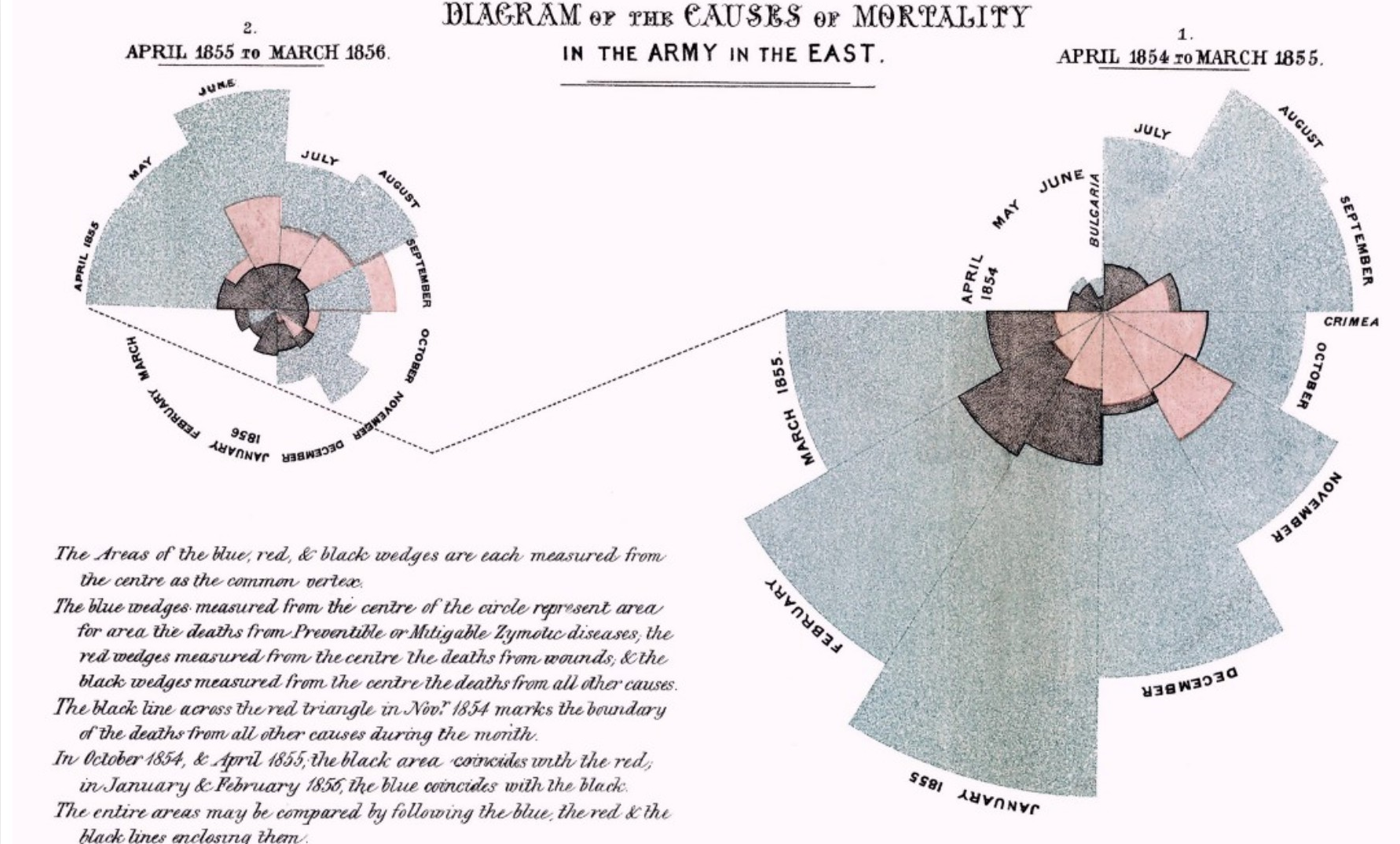Causes of Mortality in the Army of the East — Florence Nightingale (Source: https://github.com/dipanjanS/art_of_data_visualization)

# Multivariate Analysis

## Visualizing data in Three Dimensions (3-D)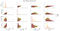Visualizing three-dimensional data with scatter plots and hue (color)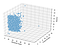Visualizing three-dimensional numeric data by introducing the notion of depth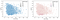Using the notion of size or hue for representing continuous data in 3-D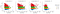Using the notion of facets for representing continous data in 3-D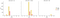Visualizing three-dimensional categorical data by introducing the notion of hue and facets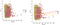Visualizing mixed attributes in three-dimensions leveraging scatter plots and the concept of hue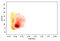Visualizing mixed attributes in three-dimensions leveraging kernel density plots and the concept of hue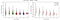Visualizing mixed attributes in three-dimensions leveraging split violin plots and the concept of hue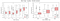Visualizing mixed attributes in three-dimensions leveraging box plots and the concept of hue

## Visualizing data in Four Dimensions (4-D)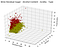Visualizing data in four-dimensions leveraging scatter plots and the concept of hue and depth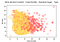Visualizing data in four-dimensions leveraging bubble charts and the concept of hue and size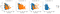Visualizing data in four-dimensions leveraging scatter plots and the concept of hue and facets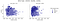Visualizing data in four-dimensions leveraging scatter plots and the concept of hue and facets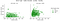Visualizing data in four-dimensions leveraging scatter plots and the concept of hue and facets

## Visualizing data in Five Dimensions (5-D)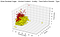Visualizing data in five-dimensions leveraging bubble charts and the concept of hue, depth and size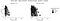Visualizing data in five-dimensions leveraging bubble charts and the concept of hue, facets and size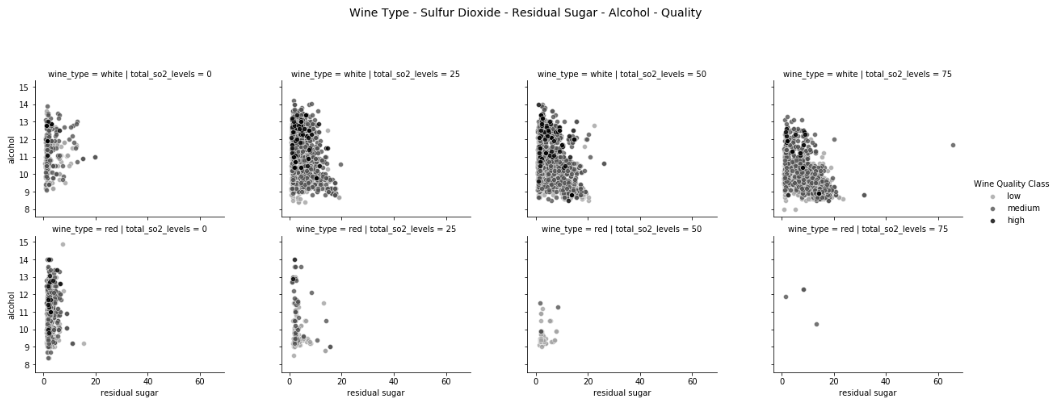Visualizing data in five-dimensions leveraging scatter plots and the concept of hue and facets

## Visualizing data in Six Dimensions (6-D)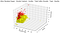Visualizing data in six-dimensions leveraging scatter charts and the concept of hue, depth, shape and size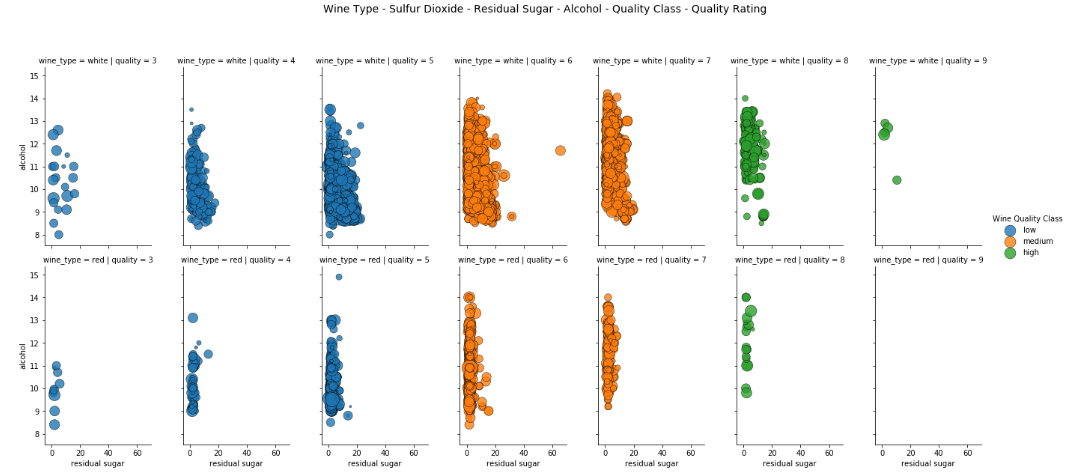Visualizing data in six-dimensions leveraging scatter charts and the concept of hue, facets and size

## Can we go higher?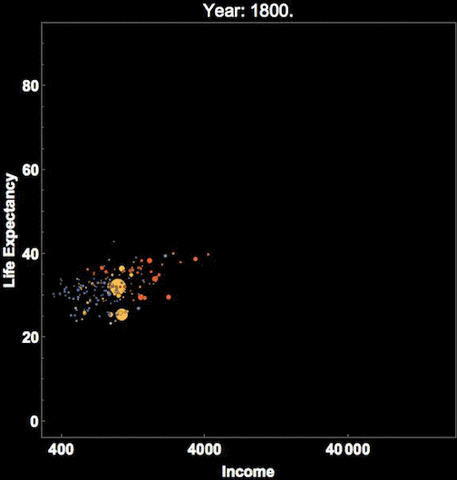Hans Rosling’s famous visualization of global population, health and economic indicators

# Conclusion

## The Startup

Get smarter at building your thing. Join The Startup’s +741K followers.

## The Startup

Get smarter at building your thing. Follow to join The Startup’s +8 million monthly readers & +741K followers.

Written by

## Dipanjan (DJ) Sarkar